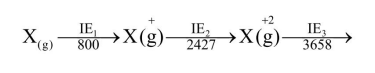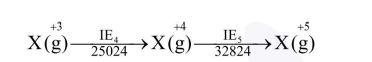# The five successive ionization enthalpies of an

Question:

The five successive ionization enthalpies of an element are $800,2427,3658,25024$ and 32824 $\mathrm{kJ} \mathrm{mol}^{-1}$. The number of valence electrons in the element is :

1. 2

2. 3

3. 4

4. 5

Correct Option: , 2

Solution:

Let suppose element $X \Rightarrow$$\mathrm{X}^{+3}$ has stable inert gas configuration as there is high jump after $\mathrm{IE}_{3}$

So valence electrons are 3